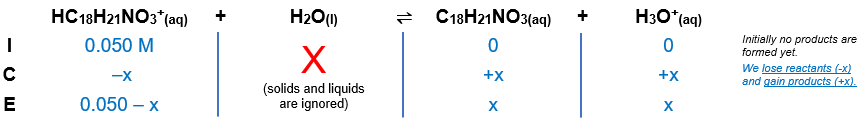# Problem: Codeine (C18H21NO3) is a narcotic pain reliever that forms a salt with HCl. What is the pH of 0.050 M codeine hydrochloride (pKb of codeine = 5.80)?

###### FREE Expert Solution

Codeine hydrochloride → salt

HC18H21NO3Cl → HC18H21NO3+ + Cl-

Equilibrium reaction:        HC18H21NO3+(aq) + H2O(l)  C18H21NO3(aq) + H3O+(aq)

***HC18H21NO3+ conjugate acid of C18H21NO3

Step 1: Construct an ICE chart for the reaction.Step 2: Write the Ka expression and calculate for Ka.

$\overline{){{\mathbf{K}}}_{{\mathbf{a}}}{\mathbf{=}}\frac{\mathbf{products}}{\mathbf{reactants}}}\phantom{\rule{0ex}{0ex}}{\mathbf{K}}_{\mathbf{a}}\mathbf{=}\frac{\mathbf{\left[}{\mathbf{C}}_{\mathbf{18}}{\mathbf{H}}_{\mathbf{21}}{\mathbf{NO}}_{\mathbf{3}}\mathbf{\right]}\mathbf{\left[}{\mathbf{H}}_{\mathbf{3}}{\mathbf{O}}^{\mathbf{+}}\mathbf{\right]}}{\left[{\mathrm{HC}}_{18}{H}_{21}{{\mathrm{NO}}_{3}}^{+}\right]}$

Solids and liquids are not included in the expression

${\mathbf{K}}_{\mathbf{b}}\mathbf{=}{\mathbf{10}}^{\mathbf{-}{\mathbf{pK}}_{\mathbf{b}}}\phantom{\rule{0ex}{0ex}}{\mathbf{K}}_{\mathbf{b}}\mathbf{=}{\mathbf{10}}^{\mathbf{-}\mathbf{5}\mathbf{.}\mathbf{80}}$

Kb = 1.5849x10–6

$\frac{{\mathbf{K}}_{\mathbf{w}}}{{\mathbf{K}}_{\mathbf{b}}}\mathbf{=}\frac{{\mathbf{K}}_{\mathbf{a}}\mathbf{·}\overline{){\mathbf{K}}_{\mathbf{b}}}}{\overline{){\mathbf{K}}_{\mathbf{b}}}}\phantom{\rule{0ex}{0ex}}{\mathbf{K}}_{\mathbf{a}}\mathbf{=}\frac{\mathbf{1}\mathbf{.}\mathbf{0}\mathbf{×}{\mathbf{10}}^{\mathbf{-}\mathbf{14}}}{\mathbf{1}\mathbf{.}\mathbf{5849}\mathbf{×}{\mathbf{10}}^{\mathbf{-}\mathbf{6}}}$

Ka = 6.3096x10–9

Step 3: Calculate the equilibrium concentrations.

${\mathbf{K}}_{\mathbf{a}}\mathbf{=}\frac{\left[\mathbf{x}\right]\left[\mathbf{x}\right]}{\left[\mathbf{0}\mathbf{.}\mathbf{050}\mathbf{-}\mathbf{x}\right]}\phantom{\rule{0ex}{0ex}}\overline{){{\mathbf{K}}}_{{\mathbf{a}}}{\mathbf{=}}\frac{{\mathbf{x}}^{\mathbf{2}}}{\mathbf{0}\mathbf{.}\mathbf{050}\mathbf{-}\mathbf{x}}}$

93% (246 ratings)###### Problem Details

Codeine (C18H21NO3) is a narcotic pain reliever that forms a salt with HCl. What is the pH of 0.050 M codeine hydrochloride (pKb of codeine = 5.80)?